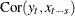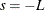# The ARIMA Procedure

### The Cross-Correlation Function

The autocorrelation and partial and inverse autocorrelation functions described in the preceding sections help when you want to model a series as a function of its past values and past random errors. When you want to include the effects of past and current values of other series in the model, the correlations of the response series and the other series must be considered.

The CROSSCORR= option in the IDENTIFY statement computes cross-correlations of the VAR= series with other series and makes these series available for use as inputs in models specified by later ESTIMATE statements.

When the CROSSCORR= option is used, PROC ARIMA prints a plot of the cross-correlation function for each variable in the CROSSCORR= list. This plot is similar in format to the other correlation plots, but it shows the correlation between the two series at both lags and leads. For example,

```   identify var=y crosscorr=x ...;
```

plots the cross-correlation function of Y and X,, forto, whereis the value of the NLAG= option. Study of the cross-correlation functions can indicate the transfer functions through which the input series should enter the model for the response series.

The cross-correlation function is computed after any specified differencing has been done. If differencing is specified for the VAR= variable or for a variable in the CROSSCORR= list, it is the differenced series that is cross-correlated (and the differenced series is processed by any following ESTIMATE statement).

For example,

```   identify var=y(1) crosscorr=x(1);
```

computes the cross-correlations of the changes in Y with the changes in X. When differencing is specified, the subsequent ESTIMATE statement models changes in the variables rather than the variables themselves.